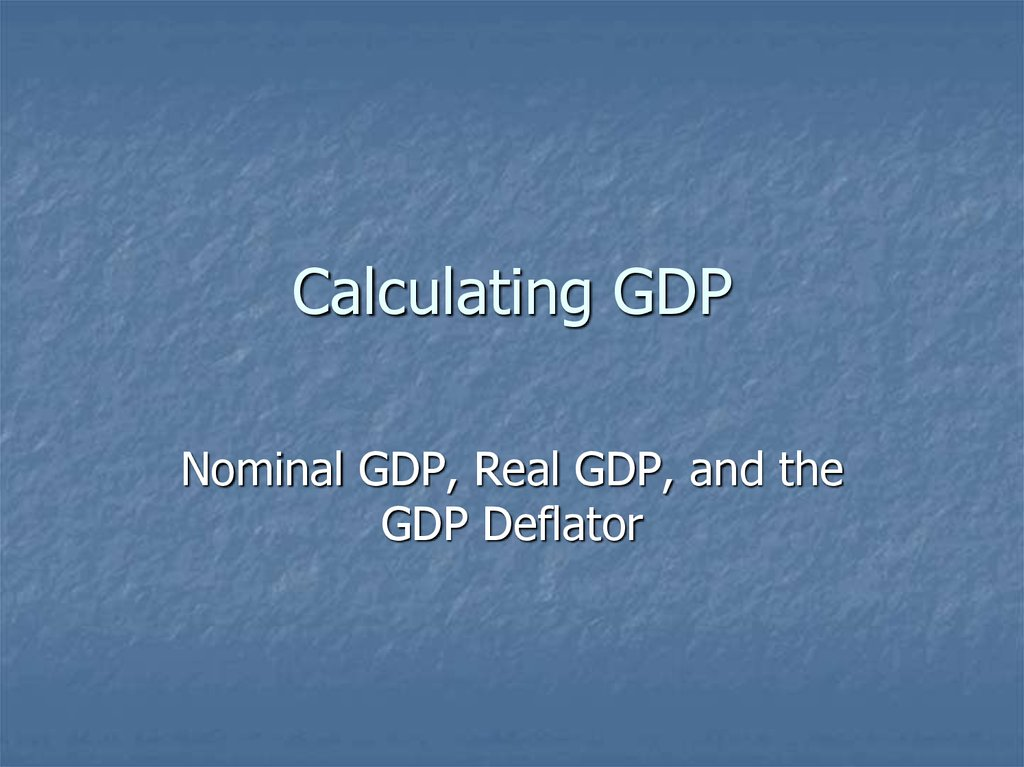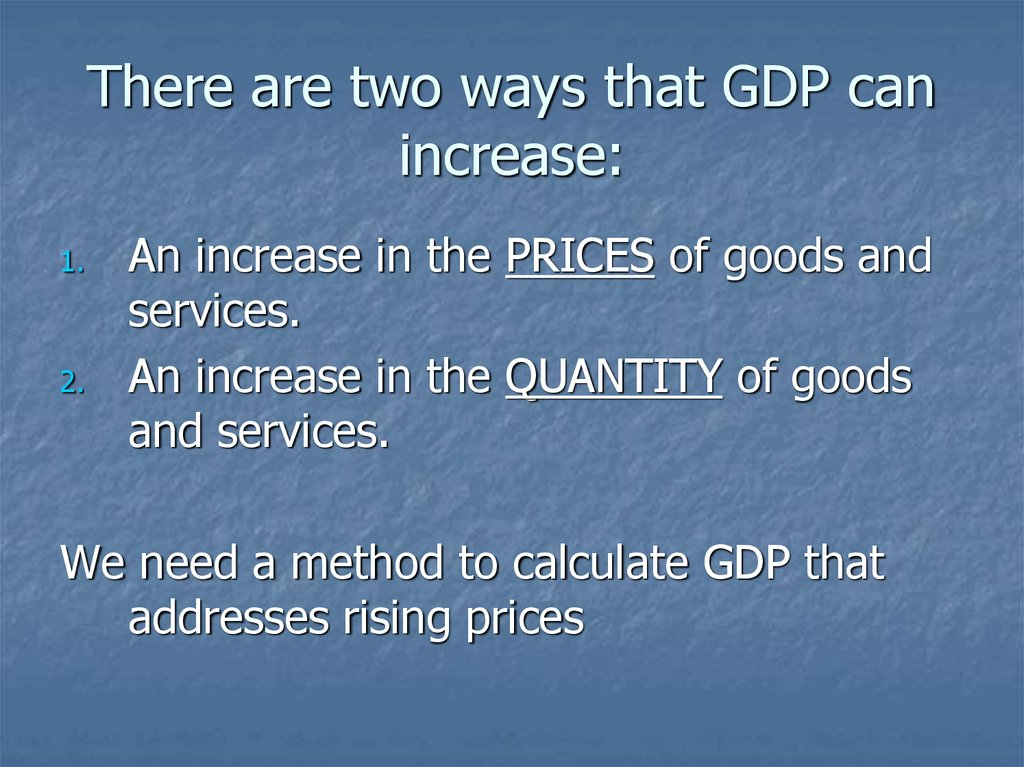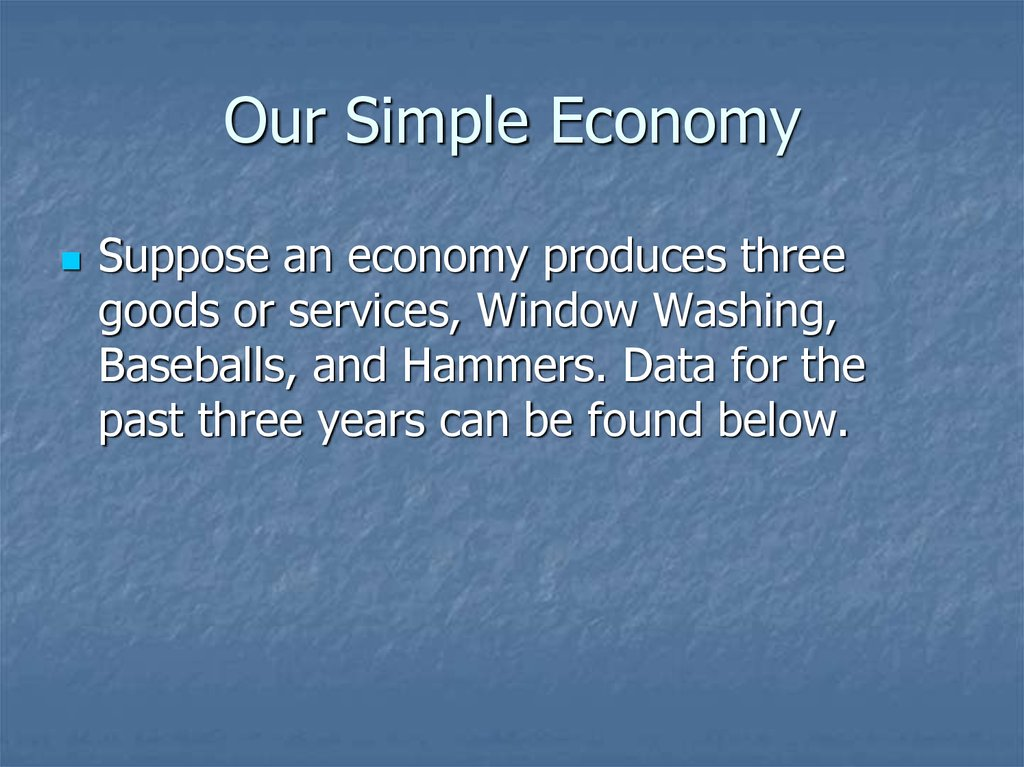# Calculating GDP. Nominal GDP, Real GDP and the GDP Deflator

## 1. Calculating GDP

Nominal GDP, Real GDP, and the
GDP Deflator

## 2. There are two ways that GDP can increase:

1.
2.
An increase in the PRICES of goods and
services.
An increase in the QUANTITY of goods
and services.
We need a method to calculate GDP that

## 3. Our Simple Economy

Suppose an economy produces three
goods or services, Window Washing,
Baseballs, and Hammers. Data for the
past three years can be found below.

## 5. Nominal GDP

Step 1: Calculate Nominal GDP (The value
of final goods and services evaluated at
current-year prices) for each year:
NGDP2006 = Q2006 x P2006
= (90 x \$50.00) Window Washing
+ (75 x \$2.00) Baseballs
+ (50 x \$30.00) Hammers
= \$6,150

## 6. Nominal GDP 2007

NGDP2007 = Q2007 x P2007
= (100 x \$60.00) Window Washing
+ (100 x \$2.00) Baseballs
+ (50 x \$25.00) Hammers
= \$7,450

## 7. Nominal GDP 2008

NGDP2008 = Q2008 x P2008
= (100 x \$65.00) Window Washing
+ (120 x \$2.25) Baseballs
+ (65 x \$25.00) Hammers
= \$8,395

## 8. Real GDP

Step 2: Calculate Real GDP (The value of
final goods and services evaluated at
base-year prices) for each year. For our
example assume 2006 is the base year.
This means that all values are in what we
call “2006 Dollars”, or “Constant Dollars”.

## 9. Real GDP

By using the prices from the base-year,
(or holding prices constant over time), we
eliminate the impact that rising prices
have on GDP, to get a measure of “Real”
economic activity.

## 10. Real GDP in 2006

RGDP2006 = Q2006 x P2006
= (90 x \$50.00) Window Washing
+ (75 x \$2.00) Baseballs
+ (50 x \$30.00) Hammers
= \$6,150
Note: For the Base-Year Nominal GDP
always equals Real GDP

## 11. Real GDP in 2007

RGDP2007 = Q2007 x P2006
= (100 x \$50.00) Window Washing
+ (100 x \$2.00) Baseballs
+ (50 x \$30.00) Hammers
= \$6,700
Note: We use “Current Quantities” and “Constant
Prices”.

## 12. Real GDP in 2008

RGDP2008 = Q2008 x P2006
= (100 x \$50.00) Window Washing
+ (120 x \$2.00) Baseballs
+ (65 x \$30.00) Hammers
= \$7,190
Note: We still use “Current Quantities” and
“Constant Prices”.

## 13. The General Formula for Calculating a Growth Rate

New _ Value Old _ Value
Percent _ Change %
100
Old _ Value
X t X t 1
Percent _ Change %
100
X t 1

## 14. Calculate the Growth Rate in Real GDP between 2006 and 2007

%Change = [(RGDP2007 – RGDP2006)/RGDP2006] x 100
%Change = [(6,700 – 6,150)/6,150] x 100
%Change = 8.94%
That is real GDP grew by 8.94% between 2006 and 2007.

## 15. Calculate the Growth Rate in Real GDP between 2007 and 2008

%Change = [(RGDP2008 – RGDP2007)/RGDP2007] x 100
%Change = [(7,190 – 6,700)/6,700] x 100
%Change = 7.31%
That is real GDP grew by 7.31% between 2007 and 2008.

## 16. The Price Level

We can use our calculations of Nominal GDP
and Real GDP to calculate the Price Level
(A measure of the average prices of goods
and services in the economy.)

## 17. The GDP Deflator

One example of a measure of the average
price level is the GDP deflator.
NGDPt
GDP _ Deflatort
100
RGDPt

## 18. Calculate the GDP Deflator for 2006

GDP Deflator2006 = (NGDP2006/RGDP2006) x 100
GDP Deflator2006 = (6,150/6,150) x 100 = 100
Note: The GDP Deflator is always equal to 100 in
the base-year.
The Price Index is “unitless”

## 19. Calculate the GDP Deflator for 2007 and 2008

GDP Deflator2007 = (NGDP2007/RGDP2007) x 100
GDP Deflator2007 = (7,450/6,700) x 100 = 111.19
GDP Deflator2008 = (NGDP2008/RGDP2008) x 100
GDP Deflator2008 = (8,395/7,190) x 100 = 116.76

## 20. The Inflation Rate

We can use the growth rate formula from
previous to calculate the Inflation Rate
(the Inflation Rate is The percentage
increase in the price level from one year
to the next.)

## 21. Calculate the Inflation Rate from 2006 to 2007

Inflation Rate Between 2006 and 2007 =
[(GDP Def.2007 – GDP Def.2006)/GDP Def.2006] x 100
Inflation Rate Between 2006 and 2007 =
[(111.19 – 100)/100] x 100 = 11.19
That is the inflation rate between 2006 and 2007
was 11.19%.

## 22. Calculate the Inflation Rate from 2007 to 2008

Inflation Rate Between 2007 and 2008 =
[(GDP Def.2008 – GDP Def.2007)/GDP Def.2007] x 100
Inflation Rate Between 2007 and 2008 =
[(116.76 – 111.19)/111.19] x 100 = 5.01
That is the inflation rate between 2007 and 2008
was 5.01%.